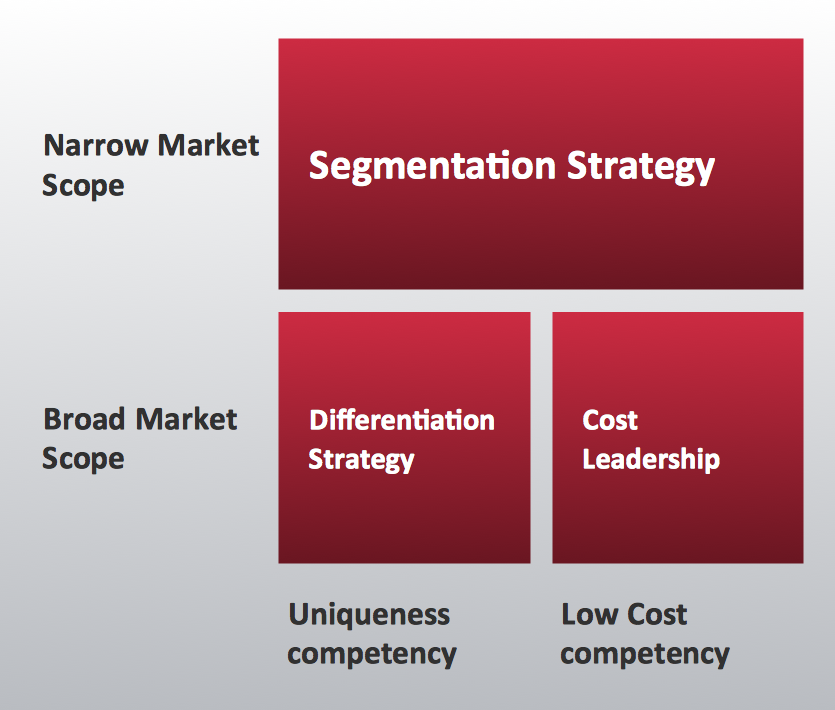This site uses cookies. By continuing to browse the ConceptDraw site you are agreeing to our Use of Site Cookies.
ConceptDraw Samples | Marketing — Matrices

# Matrices

Samples of Marketing Matrices are created with ConceptDraw DIAGRAM diagramming and vector drawing software enhanced with Matrices solution from ConceptDraw Solution Park. This solution extends ConceptDraw DIAGRAM with samples, templates and a library of design elements for drawing business matrix diagrams; including - Ansoff Matrix, BCG Matrix, Competitive Strategies Matrix, Customer Types Matrix, Deployment Chart, Feature Comparison Chart, Flow Process Chart, Porter's Value Chain Diagram, Positioning Map, Select Quality Function Deployment Matrix.

ConceptDraw DIAGRAM provides export of vector graphic multipage documents into multiple file formats: vector graphics (SVG, EMF, EPS), bitmap graphics (PNG, JPEG, GIF, BMP, TIFF), web documents (HTML, PDF), PowerPoint presentations (PPT), Adobe Flash (SWF).

## Tutorials and Solutions:

Video Tutorials: ConceptDraw Solution Park

Solutions: Matrices for ConceptDraw DIAGRAM

## Sample 1: Ansoff Matrix

Matrices sample: Ansoff matrix.

This example is created using ConceptDraw DIAGRAM diagramming and vector drawing software enhanced with Matrices solution from ConceptDraw Solution Park.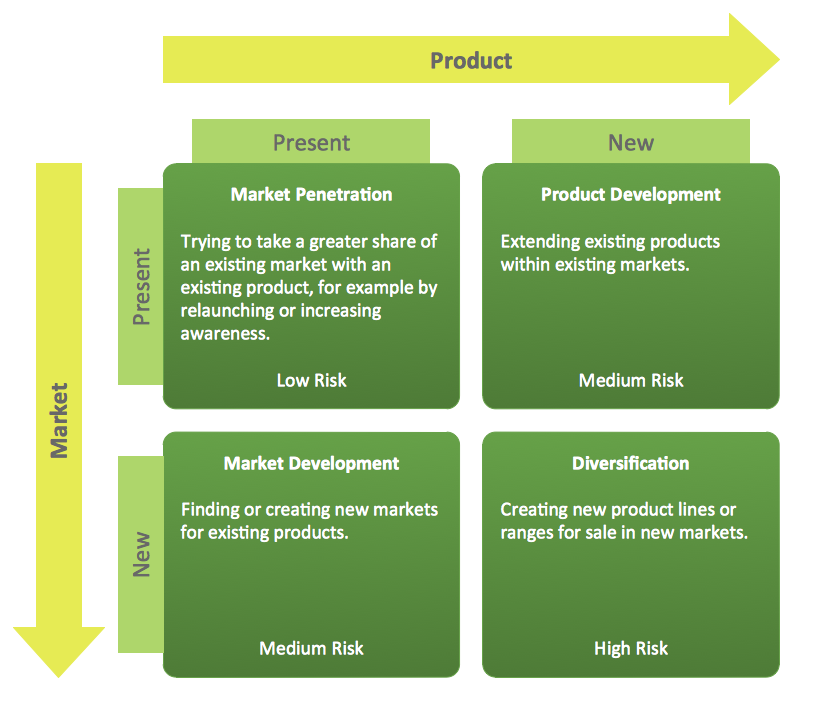## Sample 2: Matrix Diagram – Porter's Value Chain

Matrices sample: Porter's value chain.

This example is created using ConceptDraw DIAGRAM diagramming and vector drawing software enhanced with Matrices solution from ConceptDraw Solution Park.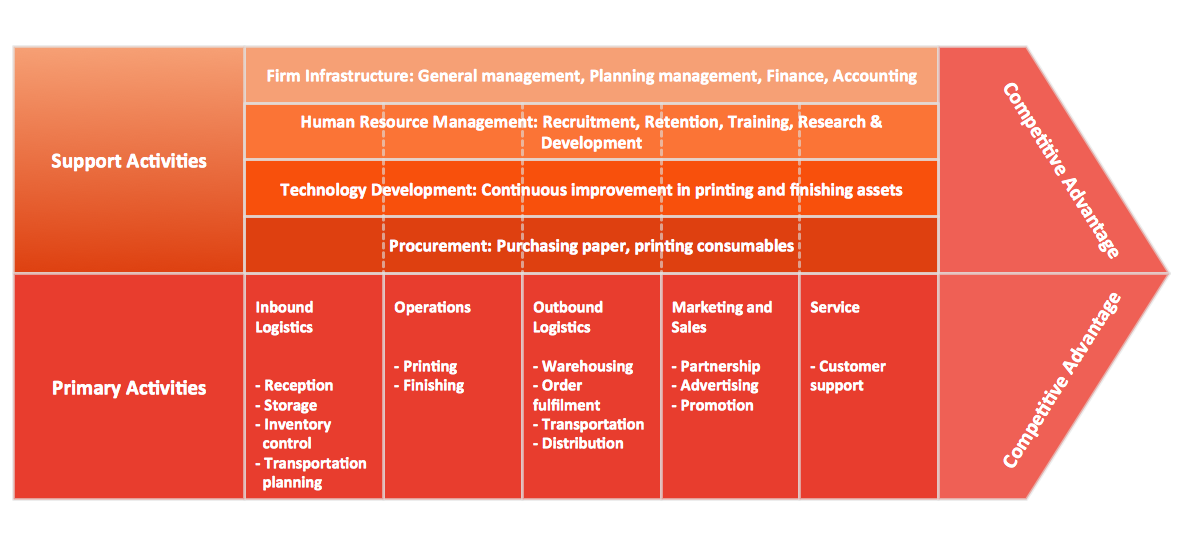## Sample 3: Matrix Diagram – Positioning Map

Matrices sample: Positioning map.

This example is created using ConceptDraw DIAGRAM diagramming and vector drawing software enhanced with Matrices solution from ConceptDraw Solution Park.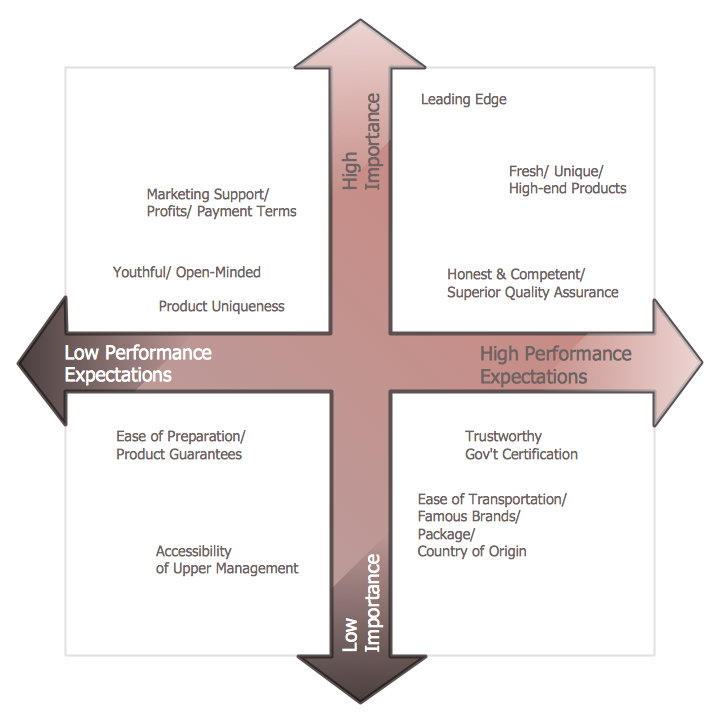## Sample 4: Boston Market Growth – Share Matrix (BCG)

Matrices sample: Boston market growth–share matrix (BCG).

This example is created using ConceptDraw DIAGRAM diagramming and vector drawing software enhanced with Matrices solution from ConceptDraw Solution Park.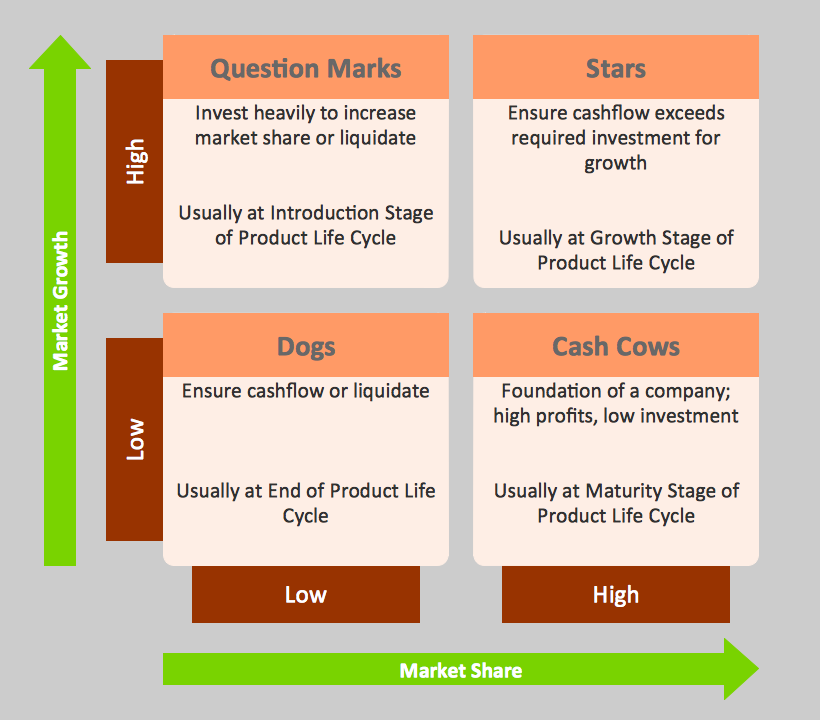## Sample 5: Feature Comparison Chart – Digital Scouting Cameras

Matrices sample: Feature comparison chart – Digital scouting cameras.

This example is created using ConceptDraw DIAGRAM diagramming and vector drawing software enhanced with Matrices solution from ConceptDraw Solution Park.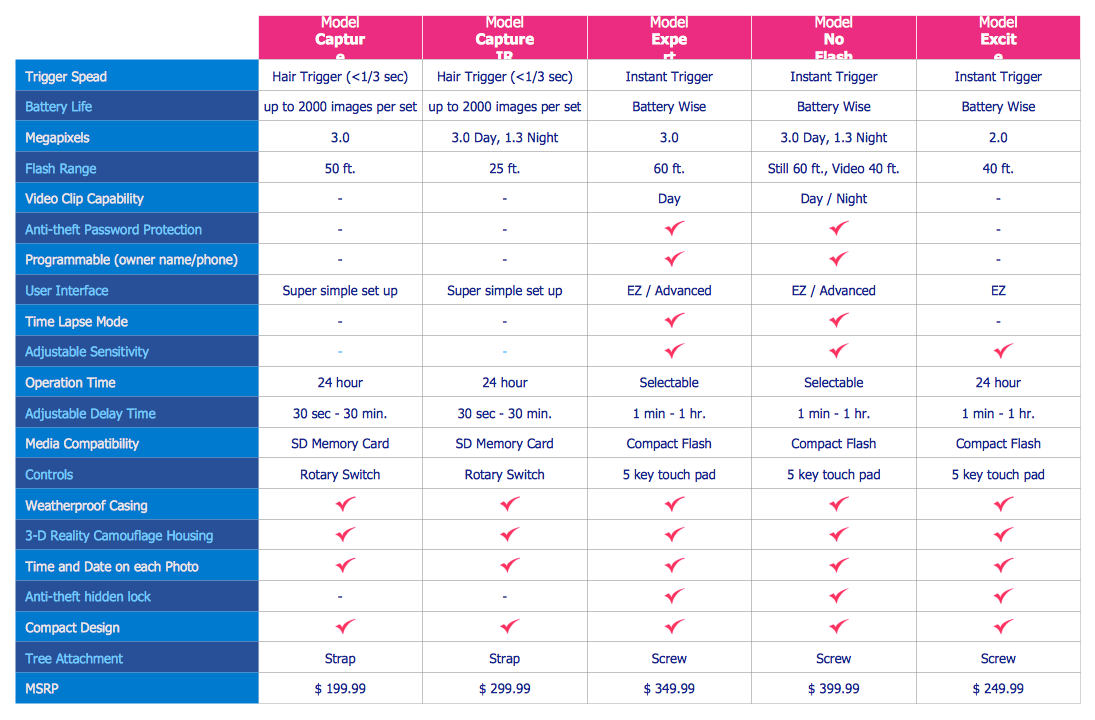## Sample 6: Competitve Strategy Matrix

Matrices sample: Competitve strategy matrix.

This example is created using ConceptDraw DIAGRAM diagramming and vector drawing software enhanced with Matrices solution from ConceptDraw Solution Park.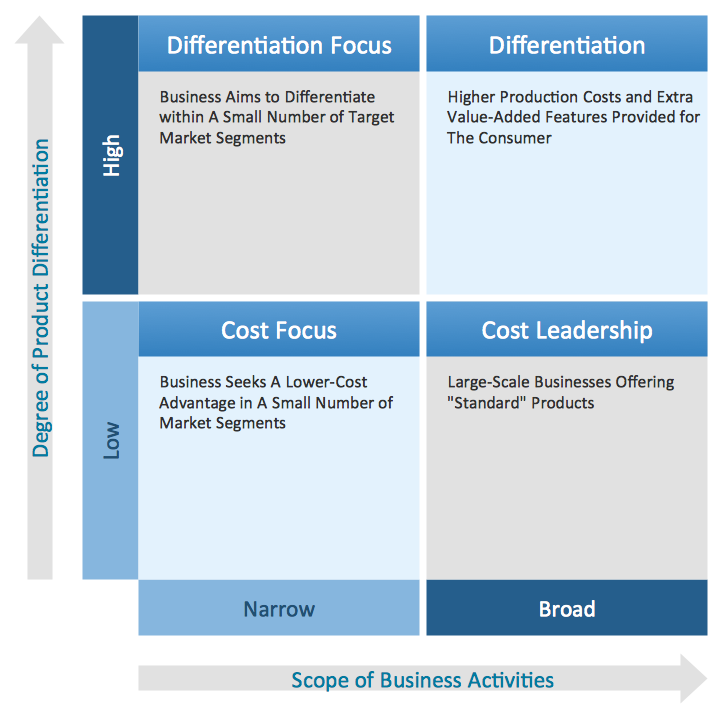## Sample 7: Customer Type Matrix Diagram

Matrices sample: Customer type matrix diagram.

This example is created using ConceptDraw DIAGRAM diagramming and vector drawing software enhanced with Matrices solution from ConceptDraw Solution Park.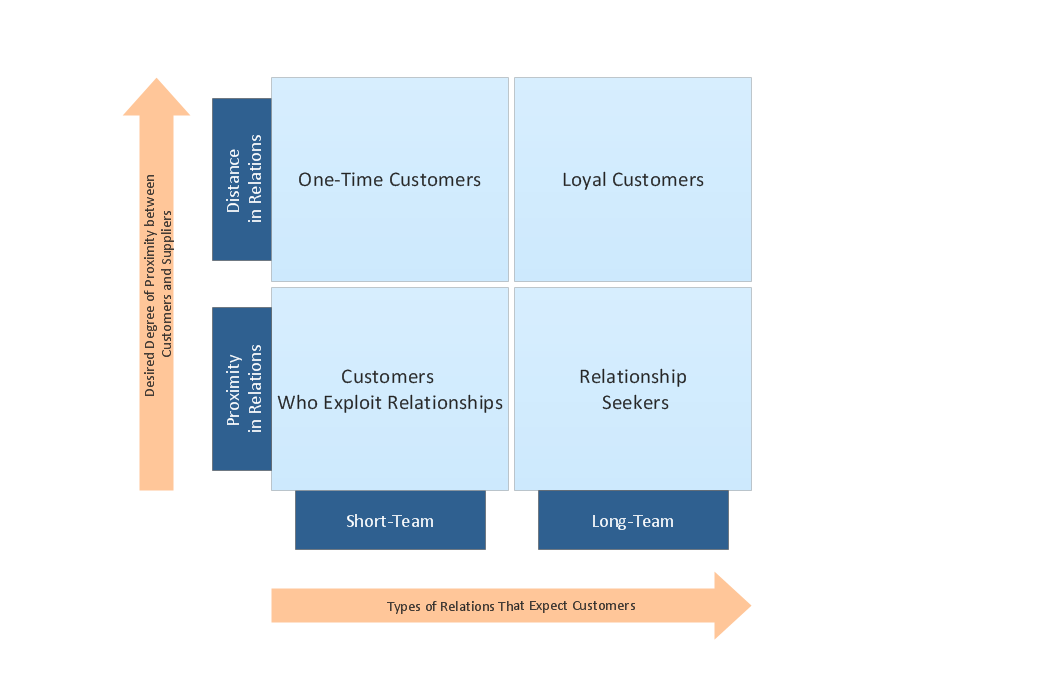## Sample 8: Matrix Diagram – Flow Process Chart

Matrices sample: Matrix diagram – Flow process chart.

This example is created using ConceptDraw DIAGRAM diagramming and vector drawing software enhanced with Matrices solution from ConceptDraw Solution Park.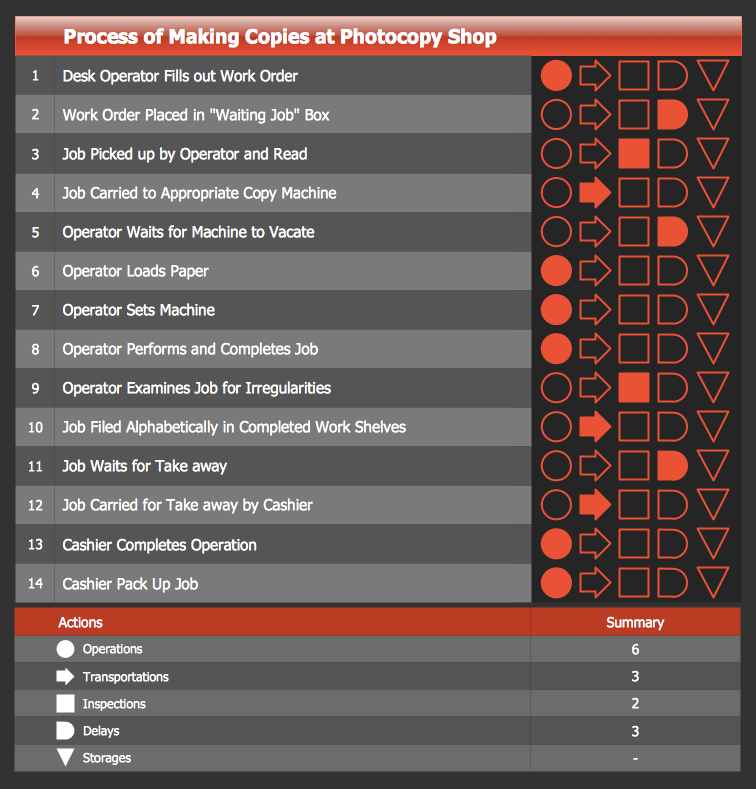## Sample 9: Quality Function Deployment Chart – Handheld Projector

Matrices sample: Quality function deployment chart – Handheld projector.

This example is created using ConceptDraw DIAGRAM diagramming and vector drawing software enhanced with Matrices solution from ConceptDraw Solution Park.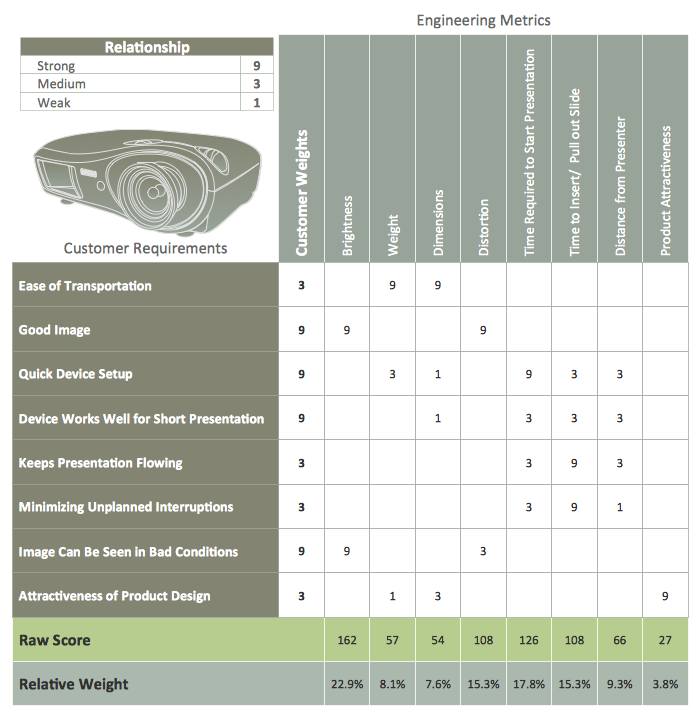## Sample 10: Matrix Diagram – Customer Types

Matrices sample: Matrix diagram – Customer types.

This example is created using ConceptDraw DIAGRAM diagramming and vector drawing software enhanced with Matrices solution from ConceptDraw Solution Park.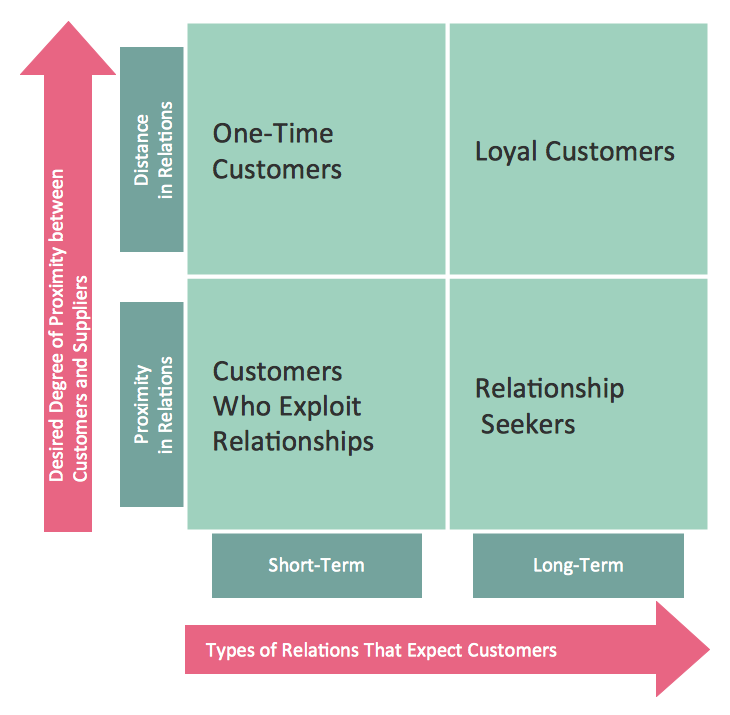## Sample 11: Matrix Diagram – Porter Generic Strategies Matrix

Matrices sample: Matrix diagram – Porter generic strategies matrix.

This example is created using ConceptDraw DIAGRAM diagramming and vector drawing software enhanced with Matrices solution from ConceptDraw Solution Park.